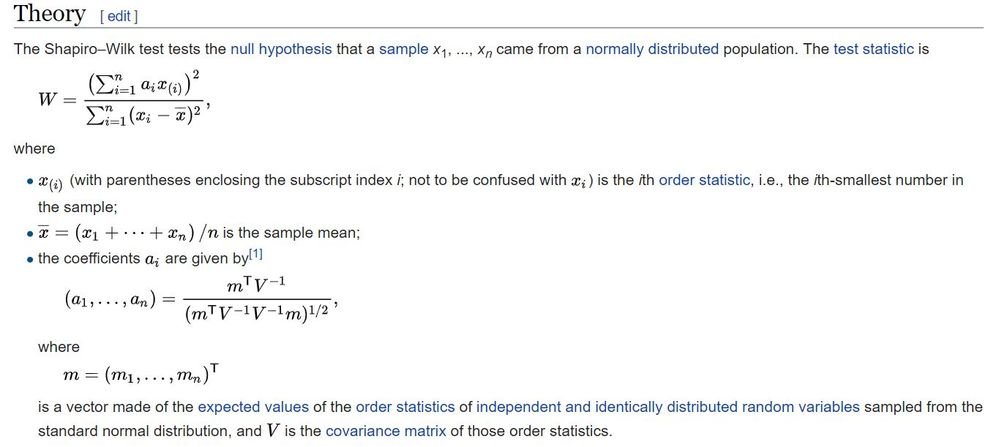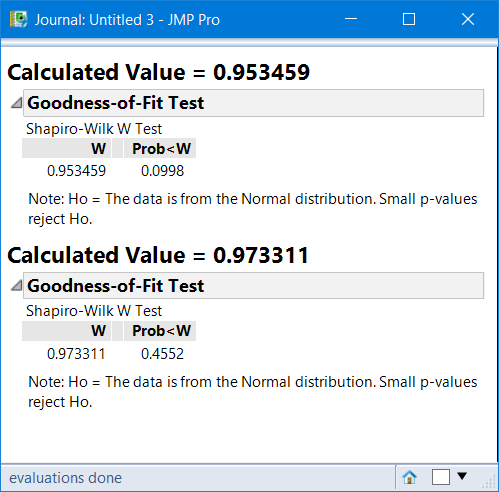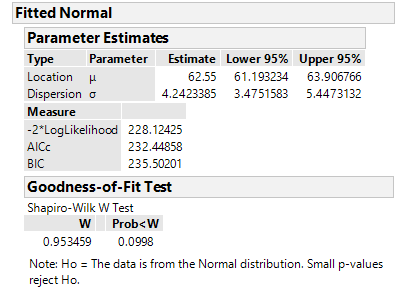Choose Language Hide Translation Bar
Highlighted

## Manual Calculation of the Shapiro-Wilk Test Statistic

Hello Everyone,

Does anyone know how to implement manual calculation of the Shapiro-Wilk test statistic in JMP?

Using JSL script and/ or column formulas?

I know that JMP natively does this calculation natively in Analyze>Distribution and then Continuous Fit>Normal>Goodness of Fit, but I'm trying to reproduce the calculated value of the test statistic in order to understand it's basis with greater depth.

There are a number of useful clues in references online, but I'm having some difficulty putting the pieces together:

https://en.wikipedia.org/wiki/Shapiro%E2%80%93Wilk_test#cite_note-8

Here's the basis for it summarized nicely from Wikepedia link above:Also some helpful clues here using MS Excel.

http://www.real-statistics.com/tests-normality-and-symmetry/statistical-tests-normality-symmetry/sha...

1 ACCEPTED SOLUTION

Accepted Solutions
Highlighted

## Re: Manual Calculation of the Shapiro-Wilk Test Statistic

@PatrickGiuliano I am not sure why you would want to do this manually, when you have JMP that can produce the statistics and you can extract it from a report.  A simulation where the sample size is the same so the weight coefficients are the same, meaning the weights are calculated once and used many times, might be a good scenario for doing this by hand/matrix.

That said, I found references for the coefficients and an algorithm to get them.  The references are reported in the attached script. Here are the results of two tests with N=40.4 REPLIES 4
Highlighted

## Re: Manual Calculation of the Shapiro-Wilk Test Statistic

Here is a link that does a step by step calculation that you might be able to use.

http://blog.excelmasterseries.com/2015/05/how-to-create-completely-automated_4.html

Jim
Highlighted

## Re: Manual Calculation of the Shapiro-Wilk Test Statistic

JMP calculates the Shapiro-Wilk's test.

• Open a data table.
• Go to Help > Statistics Index> find Shapiro-Wilk test for normality on the left, then press launch
• Select which columns of your table to be tested

The test is available in JMP PRO 12 and higher.

It is the Goodnes of Fit test for a Normal Fit. The JSL is

``````Distribution(
Continuous Distribution(
Column( :height ),
Fit Distribution( Normal( Goodness of Fit( 1 ) ) )
)
);
``````Highlighted

## Re: Manual Calculation of the Shapiro-Wilk Test Statistic

@PatrickGiuliano I am not sure why you would want to do this manually, when you have JMP that can produce the statistics and you can extract it from a report.  A simulation where the sample size is the same so the weight coefficients are the same, meaning the weights are calculated once and used many times, might be a good scenario for doing this by hand/matrix.

That said, I found references for the coefficients and an algorithm to get them.  The references are reported in the attached script. Here are the results of two tests with N=40.Highlighted

## Re: Manual Calculation of the Shapiro-Wilk Test Statistic

Thank you this is excellent.
Article Labels

There are no labels assigned to this post.